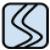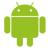## 如何给含有结构体参数的函数提供默认值

panyfx 发布于 2012/08/31 11:23

为什么80%的码农都做不了架构师？->>>struct A{

int a;

int b;

};

int function(int a, A astruct);

0```struct  A
{
int a;
int b;
};

static const A static_a = {1,2};

int funct(int i, A a=static_a)
{
printf("%d %d \n", a.a, a.b);
return 0;
}```0```struct A{
A()
: a(0),b(0)
{
}
int a;
int b;

};```0int function(int a, const A& astruct);

```int a = 0;
A a_struct;
memset(&a_struct, 0x0, sizeof(A));
function(a,a_struct);```